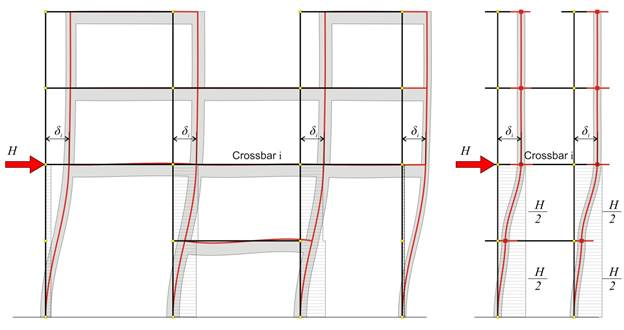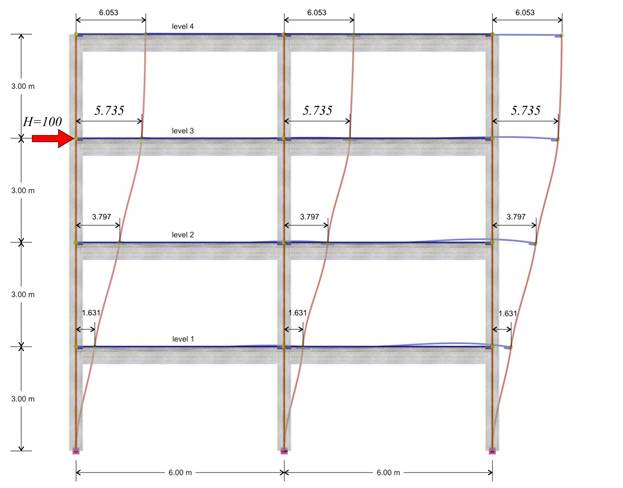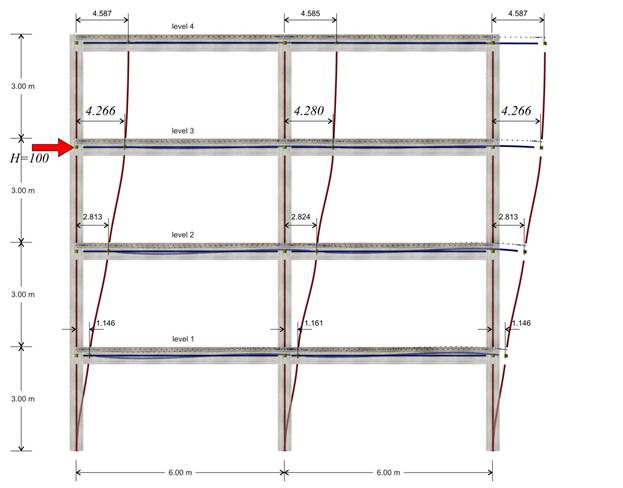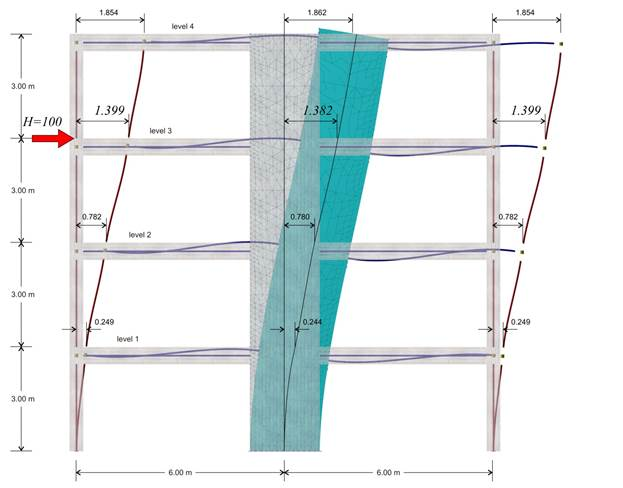# Appendix BThe structural frame
The construction
The reinforcement I
The reinforcement II
Quantity/Cost estimation
Detailing drawings
Introduction >Wind and Seismic Forces >
Structural model and Analysis
Slabs
Seismic behavour of frames
Appendix A
Appendix B
Appendix C
Appendix D
Introduction >
Modelling slabsMaterials
To be continued >
Introduction

## Stiffness of frame crossbarCommon practice frame                                                               Equivalent system

In multistorey plane frames, the quantity measuring the behaviour under horizontal seismic forces is the crossbar stiffness.

Crossbar is the horizontal part of a frame connecting columns. The crossbar is either usually a flanged beam or a slab strip.

Stiffness Ki, of crossbar i, of a plane frame is the ratio of the horizontal seismic force H acting on the crossbar, parallel to the plane of the frame, to the corresponding crossbar displacement δi, i.e. Ki=H/δi.

To compare the interstorey stiffnesses, it is very helpful to use a simpler frame type structure, with the same behaviour as the actual frame under horizontal seismic forces.

Equivalent multistorey plane system is a virtual frame consisting of the same number of storeys, with fixed-ended columns, on which when horizontal seismic force acts on a crossbar same it produces the same displacement as the one of the actual crossbar.

## Stiffness calculation of crossbar iCommon Practice Frame Frame analysis results, crossbar displacements δi and column shear forces (Stiffness of crossbar i: Ki=H/δi)   Example project : for Η=100 kN and i=2, δ2=2.589 mm → K2=H/δ2=100·103N/(2.589·10-3m)=38.6·106 N/m Equivalent System The analysis gives the same displacements, thus the same stiffness for crossbar i   Example project  < B_b1-2>: for Η=100 kN, δ2=2.589 mm → K2=38.6·106 N/m

## Equivalent multistorey frame – crossbar relative stiffness Example project < B_b1-1>Analysis of crossbar i under horizontal force H Example: for Η=100 kN and i=2, δ2=2.589 mm Analysis of crossbar i-1 under horizontal force H Example: for Η=100 kN and i-1=1, δ1=0.936mm The relative stiffness of crossbar i is equal to ΚZ,i=H/( δi-δi-1). Example: ΚΖ,2=100.00×103N/[(2.589-0.936)×10-3m=60.5 N/m

The equivalent frame may comprise one, two, or more vertical columns and should result in the same displacements as the actual frame. It is easier to perceive this equivalence using an one-bay frame composed of equivalent fixed-ended columns and eventual segmented crossbars.

Relative stiffness KRi, of crossbar i, of a multistorey plane frame is the ratio of the horizontal seismic force Η acting on crossbar i, to the relative displacement between the crossbar i and the underlying crossbar i-1, when identical force Ηacts on the latter, i.e. KRi=H/(δi-δi-1).

The stiffnesses Κi and the relative stiffnesses ΚZi of all crossbars are calculated tabulating the above procedure

 Crossbar i δo,i Κi δi-δi-1 ΚZi Equivalent cross-sections Mm ×106 N/m mm ×106 N/m mm/mm 5 6.6789 14.97 2.810(*) 35.59 418/418 4 6.1173 16.35 2.248 44.48 443/443 3 3.869 25.85 1.280 78.13 429/429 2 2.589 38.62 1.653 60.50 401/401 1 0.936 106.84 0.936 106.84 464/464

## Relative stiffness of crossbar column

 Crossbar i ΚZ Vi,1 ΚZi,1 Vi,2 ΚZi,2 Vi,3 ΚZi,3 Vi,4 ΚZi,4 Σ(Vi) ×106 N/m kN ×106 N/m kN ×106 N/m kN ×106 N/m kN ×106 N/m 5 35.59 - - - - 75.8 27.0 24.2 8.6 100.00 4 44.48 38.8 17.3 61.2 27.2 - - - - 100.00 3 78.13 18.6 14.5 36.6 28.6 34.9 27,3 9.9 7.7 100.00 2 60.50 9.0 5.4 44.2 26.7 43.6 26.4 3.2 1.9 100.00 1 106.84 4.0 4.3 47.3 50.5 47.3 50.5 1.4 1.5 100.00

The shear forces Vi,j carried by the column j of level i and
the corresponding relative stiffnesses
ΚZi,j

Relative stiffness of crossbar column of multistorey plane frame is the ratio of its shear force to the force H multiplied by the relative crossbar stiffness KRi.

Important: The examination of the behaviour of multistorey frames, beyond its educational purpose is also the basis of the assessment of the multistorey space frames, which function along both plane frame directions x and y. Of course, the rotation factor, considered in Appendix D, is also involved in the space frame analysis.

## Effect of slabs on stiffnesses using finite elements

Indicatively a four-storey frame of 3.0 m high columns is analysed. Each floor comprises columns of 400/400 cross-section, beams of 6.0 m span and 300/500 cross-section and slabs of 150 mm thickness and 1.0 m width at each side of beam faces.

Two different analyses are performed in the project <B_b4>. Their resulting displacements are presented in the two following pages, while the results and conclusions are presented in the third. In both analyses all the stiffness factors are taken equal to 1.0, rigid bodies and shear effect are taken into account. The analyses results are compared by means of the crossbar stiffness at the third level.

## First analysis: frame type structure, modelled with membersThe deformation of the structure

First analysis: a plane frame is analysed, where the influence of slabs is accounted for assuming the effective flange width in accordance with EC2. In frame analysis, beams and columns are usually modelled with members. The influence of slabs is taken into account by adding a beff wide flange to the rectangular beam, and a flanged beam is formed, usually of T section.

First analysis results: as shown in the previous pages, the displacement of the crossbar at the 3rd level, due the horizontal force H=100 kN, is δ3,1=5.735 mm, therefore its stiffness isK3,1=H/δ3,1=100.0·103N/(5.735·10-3m)=17.4·106 N/m.

The following elastic displacements δi,1 and stiffnesses Ki,1 are obtained from similar analyses applying force H=100 kN separately on each floor i.

4th level: H=100 kN, δ4,1=8.051 mm, K4,1=12.4·106 N/m

3rd level: H=100 kN, δ3,1=5.735 mm, K3,1=17.4·106 N/m

2nd level: H=100 kN δ2,1=3.500 mm, K2,1=28.6·106 N/m

1st level: H=100 kN, δ1,1=1.358 mm, K1,1=73.6·106 N/m

## 2η επίλυση: Πλαισιακός φορέας με προσομοίωση ραβδωτών και επιφανειακών πεπερασμένων στοιχείωνThe deformation of the structure

Second analysis: frame type structure, members and finite elements,
under a horizontal force H acting at the 3rd level.

Second analysis results: as shown in the previous page, the displacement of the crossbar at the 3rd level, due to horizontal force H=100.0 kN, is δ3,2=4.266 mm, therefore its stiffness yields K3,2=H/δ3,2=100.0·103N/(4.266·10-3m)=23.4·106 N/m.

The following elastic displacements δi,1 and stiffnesses Ki,1 are obtained from similar analyses due to the force H=100 kN separately on each floor i.

4th level: H=100 kN, δ4,2=6.073 mm, K4,2=16.5·106 N/m

3rd level: H=100 kN, δ3,2=4.266 mm, K3,2=23.4·106 N/m

2nd level: H=100 kN δ2,2=2.528 mm, K2,2=39.6·106 N/m

1st level: H=100 kN, δ1,2=0.886 mm, K1,2=112.9·106 N/m

Conclusion: The frame stiffness derived from the finite element analysis is higher.

## Effect of walls on stiffnesses using finite elements

As the effect of slabs on stiffness was examined in the previous paragraph, in order to obtain comparable results, a two-bay frame without slabs is considered next.
Indicatively a four-storey frame with 3.0 m high columns is analysed. Each floor comprises two columns of 400/400 cross-section, one wall of 2000/300 cross-section and two beams of 6.0 m span and 300/500 cross-section.
Columns and beams are modelled with members, while walls are modelled with members and rigid bodies at their tops, or with triangular finite elements.

## First analysis: plane frame, whose walls are modelled with members and rigid bodies at their topsThe deformation of the structure

First analysis results: The displacement of the crossbar at the 3rd level, due to horizontal force H=100 kN, is δ3,1=1.552 mm, therefore its stiffness is K3,1=H/δ3,1=100.0·103N/(1.552·10-3m)=64.4·106 N/m.

The following elastic displacements δi,1 and stiffnesses Ki,1 are obtained from similar analyses applying force H=100 kN separately on each floor i.

4η level: H=100 kN, δ4,1=3.033 mm, K4,1=33.0·106 N/m

3rd level: H=100 kN, δ3,1=1.552 mm, K3,1=64.4·106 N/m

2η level: H=100 kN δ2,1=0.629 mm, K2,1=159.0·106 N/m

1η level: H=100 kN, δ1,1=0.146 mm, K1,1=685.0·106 N/m

## Second analysis: Dual type structure, modelled with members and triangular finite elements (The finite element size is taken equal to 0.30 m across the slab surfaced and 0.15 m across the slab perimeter)The deformation of the structure

Second analysis results: The displacement of the crossbar at the 3rd level, due to horizontal force H=100.0 kN, is δ3,2=1.399 mm, therefore its stiffness yields K3,1=H/δ3,1=100.0·103N/(1.399·10-3m)=71.5·106 N/m.

The following elastic displacements δi,1 and stiffnesses Ki,1 are obtained from similar analyses applying force H=100 kN separately on each floor i.

4th level: H=100 kN, δ4,2=2.783 mm, K4,2=35.9·106 N/m

3rd level: H=100 kN, δ3,2=1.399 mm, K3,2=71.5·106 N/m

2nd level: H=100 kN δ2,2=0.543 mm, K2,2=184.0·106 N/m

1st level: H=100 kN, δ1,2=0.117 mm, K1,2=855.0·106 N/m

In the example considered, at the 3rd level, the stiffness obtained using finite element modelling is (71.5-64.4)/64.4=11% higher, while at the 4th, 2nd and 1st level 9%, 16% and 25% higher, respectively.

Conclusion: The frame stiffness slightly differs when walls are modelled with two-dimensional finite elements.